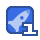# gjk C++应用代码（碰撞问题）

4星（超过85%的资源）50收藏

GJK计算碰撞代码的应用 //----------------------------------------------------------------------------- // Torque 3D // Copyright (C) GarageGames.com, Inc. // // The core algorithms in this file are based on code written // by G. van den Bergen for his interference detection library, // "SOLID 2.0" //----------------------------------------------------------------------------- #include "core/dataChunker.h" #include "collision/collision.h" #include "sceneGraph/sceneObject.h" #include "collision/convex.h" #include "collision/gjk.h" //---------------------------------------------------------------------------- static F32 rel_error = 1E-5f; // relative error in the computed distance static F32 sTolerance = 1E-3f; // Distance tolerance static F32 sEpsilon2 = 1E-20f; // Zero length vector static U32 sIteration = 15; // Stuck in a loop? S32 num_iterations = 0; S32 num_irregularities = 0; //---------------------------------------------------------------------------- GjkCollisionState::GjkCollisionState() { a = b = 0; } GjkCollisionState::~GjkCollisionState() { } //---------------------------------------------------------------------------- void GjkCollisionState::swap() { Convex* t = a; a = b; b = t; CollisionStateList* l = mLista; mLista = mListb; mListb = l; v.neg(); } //---------------------------------------------------------------------------- void GjkCollisionState::compute_det() { // Dot new point with current set for (int i = 0, bit = 1; i < 4; ++i, bit <<=1) if (bits & bit) dp[i][last] = dp[last][i] = mDot(y[i], y[last]); dp[last][last] = mDot(y[last], y[last]); // Calulate the determinent det[last_bit][last] = 1; for (int j = 0, sj = 1; j < 4; ++j, sj <<= 1) { if (bits & sj) { int s2 = sj | last_bit; det[s2][j] = dp[last][last] - dp[last][j]; det[s2][last] = dp[j][j] - dp[j][last]; for (int k = 0, sk = 1; k < j; ++k, sk <<= 1) { if (bits

...展开详情守钱 代码很简单,也没有优化,但是能看清楚gjk的基本逻辑,非常不错
2016-05-19fatislord 只有头文件和源文件，不知道怎么用。不过还是感谢分享
2015-08-13

2014-06-05dhull 只有头文件和源文件，不知道怎么用。不过还是感谢分享。
2014-03-07

• 1
资源
• 0
粉丝
•等级gjk C++应用代码（碰撞问题） 15积分/C币 立即下载
1/0